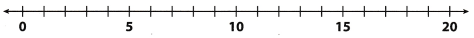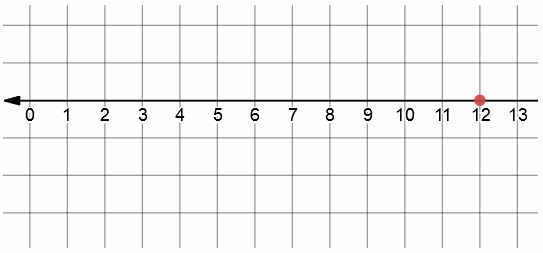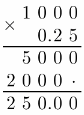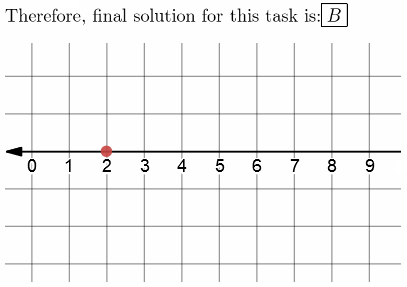Refer to our Texas Go Math Grade 6 Answer Key Pdf to score good marks in the exams. Test yourself by practicing the problems from Texas Go Math Grade 6 Module 1 Answer Key Integers.

Compare. Write <, >, or =.

Question 1.
471 __________ 468
Number 471 is greater than 168
Therefore, we write:
471 > 468

Question 2.
5,005 __________ 5,050
Number 5,005 is smaller than 5,050
Therefore, we write:
5. 005 < 5,050

Question 3.
398 __________ 389
Number 398 is greater than 389
Therefore, we write:
398 > 389

Question 4.
10,973 __________ 1099
Number 10, 973 is smaller than 10,999
Therefore, we write:
10, 973 < 10,999

Question 5.
8,471 __________ 9,001
Number 8,471 is smaller than 9,001
Therefore, we write:
8, 471 < 9,001

Question 6.
108 __________ 95
Number 108 is greater than 95
Therefore, we write:
108 > 95

Order the numbers from greatest to least.

Question 7.
156; 87; 177; 99
Number 177 is greater than 156 which is greater than 99 which is greater than 87
Therefore, we write:
177 > 156 > 99 > 87

Question 8.
591; 589; 603; 600
Number 603 is greater than 600 which is greater than 591 which is greater than 589
Therefore, we write:
603 > 600 > 591 > 589

Question 9.
2,650; 2,605; 3,056; 2,088
Number 3,056 is greater than 2,650 which is greater than 2,605 which is greater than 2,088
Therefore, we write:
3, 056 > 2. 650 > 2.605 > 2. 088

Question 10.
1,037; 995; 10,415; 1,029
Number 10,415 is greater than 1,037 which is greater than 1,029 which is greater than 995
Therefore, we write:
10,115 > 1,037 > 1, 029 > 995

Graph each number on the number line.Question 11.
12Question 12.
20
Here, we know weight of 1 hamburger’s meat needs.
If we want to know the weight of 1000 hamburgers’s meat needs, we need to multiply it!Question 13.
2
Firstly, we know that the building has 4 floors with 12 apartments on each!
That means that building has:
4 ∙ 12 = 48 apartments.
If we know that each floor has 3 apartments which are not one-bedroom ones, that implies
That there are:
4 ∙ 3 = 12 apartments.
which are not one-bedroom ones! Now we subtract values and get:
48 – 12 = 36
apartments with one bedroom!
Now, we know that every expression that does not give 48, when evaluated, is wrong!
Therefore, final solution for this task isQuestion 14.
9
Here, we calculate the content of parenthesis first!
So. we get:
900 + 60000 + 200000 + 80 = 260980
Note that i automatically calculated that because when we multiply with such numbers.
all we do is adding certain number of 0 to time right of the last digit!Visualize Vocabulary
Use the ✓ words to complete the chart. Write the correct vocabulary word next to the symbol.Understand Vocabulary

Complete the sentences using the preview words.

Question 1.
An ___________ is a statement that two quantities are not equal.